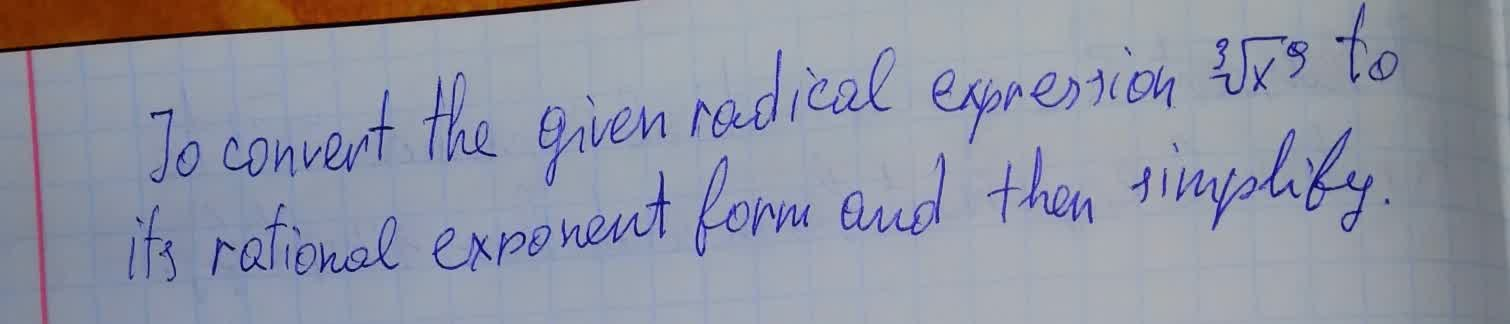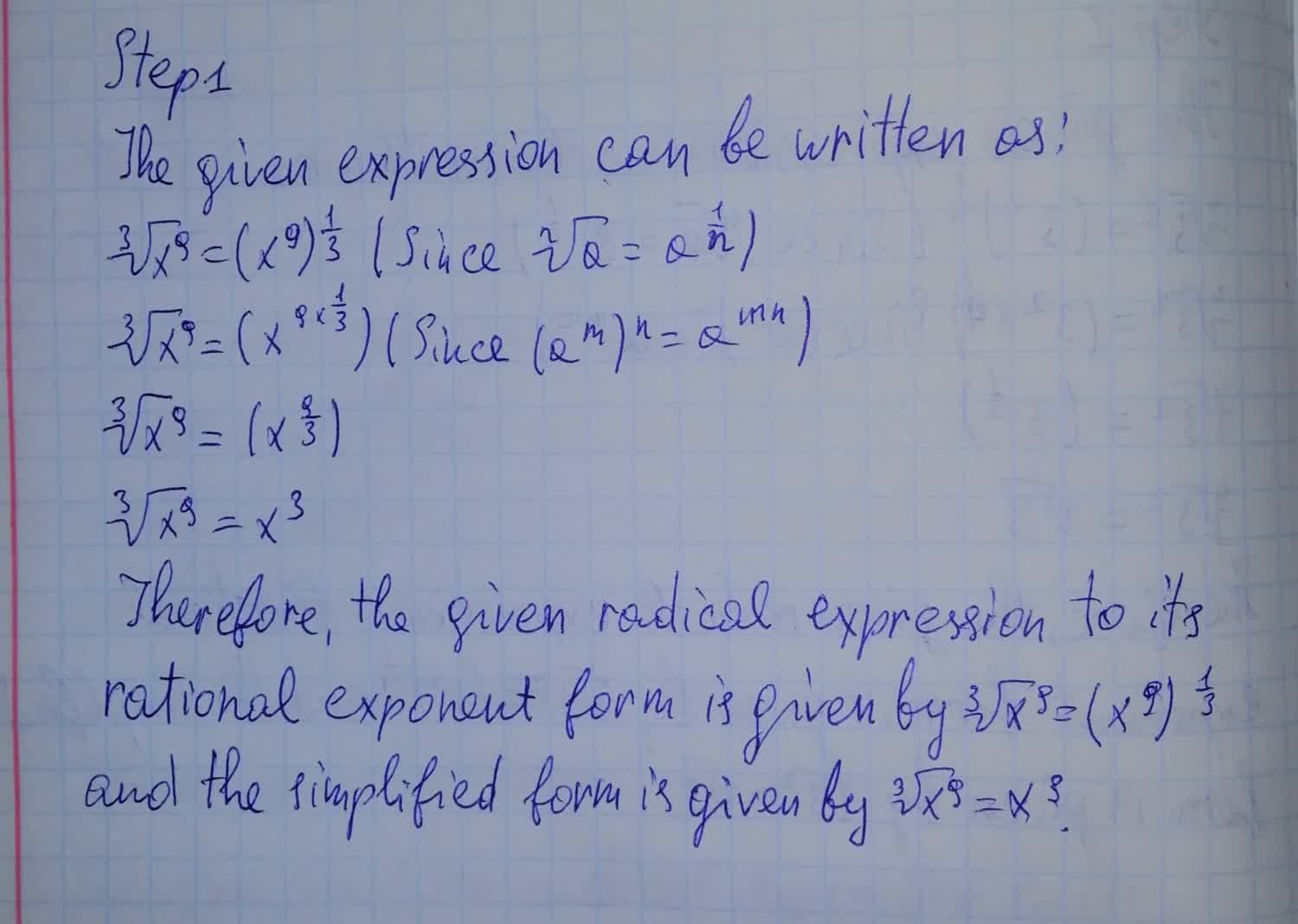# To convert the given radical expression \sqrt{x^9} to its rational exponent form and then simplifyTrent Carpenter 2021-08-12 Answered

To convert the given radical expression $$\sqrt{x^9}$$ to its rational exponent form and then simplify• Questions are typically answered in as fast as 30 minutes

### Plainmath recommends

• Get a detailed answer even on the hardest topics.
• Ask an expert for a step-by-step guidance to learn to do it yourself.Ezra Herbert